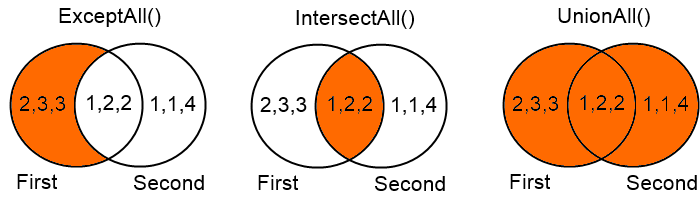## Except, intersect and union without distinct

Immaging that you have a customer that should pay you €10, €10, €20, €30, €30. He already paid you €10, €20. You need to know what he still needs to pay you. How will C#/LINQ helps you to give the answer?

``````
var all = new[] { 10, 10, 10, 20, 30, 30 };
var paid = new[] { 10, 20 };
var stillNeedToPay = all.Except(paid); // 30

```
```

Now he does not have to pay the the other 2 x €10 and only one of the two €30. This because Except is a set operation, just like the SQL set operation. This applies an implicit DISTINCT filter, unless you don't tell SQL to return ALL. But in LINQ there is no ALL variant. The same story for the set operations Intersect and Union

That is why I created an All-variant that will do the same as Except All. All you need to do is this:

``````
var stillNeedToPay = all.ExceptAll(paid); // 10, 10, 30, 30

```
```

Here you see the difference with the regular and the all-variants:

``````
var first = new[] { 1, 2, 2, 2, 3, 3 };
var second = new[] { 1, 1, 1, 2, 2, 4 };

// Regular
var except = first.Except(second); // 3
var except2 = second.Except(first); // 4
var intersect = first.Intersect(second); // 1, 2
var union = first.Union(second); // 1, 2, 3, 4

// All-variants
var exceptAll = first.ExceptAll(second); // 2, 3, 3
var exceptAll2 = second.ExceptAll(first); // 1, 1, 4
var intersectAll = first.IntersectAll(second); // 1, 2, 2
var unionAll = first.Union(second); // 1, 2, 2, 2, 3, 3, 1, 1, 4

```
```

This is a graphical view of what ExceptAll(), IntersectAll() and UnionAll() are doing:The code to find out the ExceptAll results could be as short as this:

``````
var listsecond = second.ToList();
var exceptAllresult = first.Where(i => !listsecond.Remove(i));

```
```

But that is nasty (Remove method in LINQ statement) and terribly slow with large collections, so I created a collection to remove values faster:

``````
public class ValueCounter<T> : IEnumerable<KeyValuePair<T, int>>
{
private int _nullCount;

public ValueCounter(IEnumerable<T> values,
IEqualityComparer<T> comparer)
{
// double ? operator don't works in syntax highlighter,
// so I used the ?: operator to display correct
_valueCounter = new Dictionary<T, int>
(comparer != null ? comparer : EqualityComparer<T>.Default);
if (values == null)
return;
foreach (var value in values)
{
}
}

public ValueCounter(IEqualityComparer<T> comparer)
: this(null, comparer)
{
}

public ValueCounter(IEnumerable<T> values)
: this(values, null)
{
}

public ValueCounter()
: this(null, null)
{
}

{
if (value == null)
{
_nullCount++;
}
else
{
int count;
if (_valueCounter.TryGetValue(value, out count))
{
// Double lookup is faster then creating a StrongBox
_valueCounter[value] = count + 1;
}
else
{
}
}
}

public bool Remove(T value)
{
if (value == null)
{
if (_nullCount > 0)
{
_nullCount--;
return true;
}
}
else
{
int count;
if (_valueCounter.TryGetValue(value, out count))
{
if (count == 0)
{
return false;
}
// Double lookup is faster then creating a StrongBox
_valueCounter[value] = count - 1;
return true;
}
}
return false;
}

public int GetCount(T value)
{
if (value == null)
{
return _nullCount;
}
int result;
_valueCounter.TryGetValue(value, out result);
return result;
}

public IEnumerator<KeyValuePair<T, int>> GetEnumerator()
{
return _valueCounter.GetEnumerator();
}

IEnumerator IEnumerable.GetEnumerator()
{
return GetEnumerator();
}
}

```
```

Finally the faster implementation of the all-variants:

``````
public static IEnumerable<TSource> ExceptAll<TSource>(
this IEnumerable<TSource> first,
IEnumerable<TSource> second)
{
return ExceptAll(first, second, null);
}

public static IEnumerable<TSource> ExceptAll<TSource>(
this IEnumerable<TSource> first,
IEnumerable<TSource> second,
IEqualityComparer<TSource> comparer)
{
if (first == null) { throw new ArgumentNullException("first"); }
if (second == null) { throw new ArgumentNullException("second"); }

return ExceptAllImplementation(first, second, comparer);
}

private static IEnumerable<TSource> ExceptAllImplementation<TSource>(
this IEnumerable<TSource> first,
IEnumerable<TSource> second,
IEqualityComparer<TSource> comparer)
{

var valueCounter = new ValueCounter<TSource>(second, comparer);

// Do not convert to Where, this enumerates wrong the second time
foreach (TSource s in first)
{
if (!valueCounter.Remove(s))
{
yield return s;
}
}
}

public static IEnumerable<TSource> IntersectAll<TSource>(
this IEnumerable<TSource> first,
IEnumerable<TSource> second)
{
return IntersectAll(first, second, null);
}

public static IEnumerable<TSource> IntersectAll<TSource>(
this IEnumerable<TSource> first,
IEnumerable<TSource> second,
IEqualityComparer<TSource> comparer)
{
if (first == null) { throw new ArgumentNullException("first"); }
if (second == null) { throw new ArgumentNullException("second"); }

return IntersectAllImplementation(first, second, comparer);
}

private static IEnumerable<TSource> IntersectAllImplementation<TSource>(
this IEnumerable<TSource> first,
IEnumerable<TSource> second,
IEqualityComparer<TSource> comparer)
{

var valueCounter = new ValueCounter<TSource>(second, comparer);

// Do not convert to Where, this enumerates wrong the second time
foreach (TSource s in first)
{
if (valueCounter.Remove(s))
{
yield return s;
}
}
}

public static IEnumerable<TSource> UnionAll<TSource>(
this IEnumerable<TSource> first,
IEnumerable<TSource> second)
{
return UnionAll(first, second, null);
}

public static IEnumerable<TSource> UnionAll<TSource>(
this IEnumerable<TSource> first,
IEnumerable<TSource> second,
IEqualityComparer<TSource> comparer)
{
if (first == null) { throw new ArgumentNullException("first"); }
if (second == null) { throw new ArgumentNullException("second"); }

var firstCache = first.ToList();
return firstCache.Concat(second.ExceptAll(firstCache, comparer));
}

```
```

The code in this post has been improved bij my former colleague Frank Bakker. Thanks as lot!